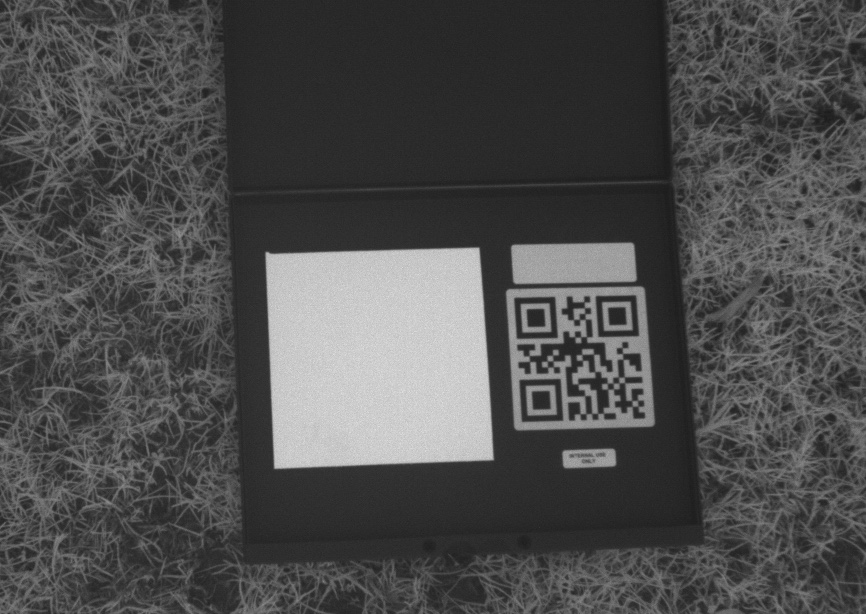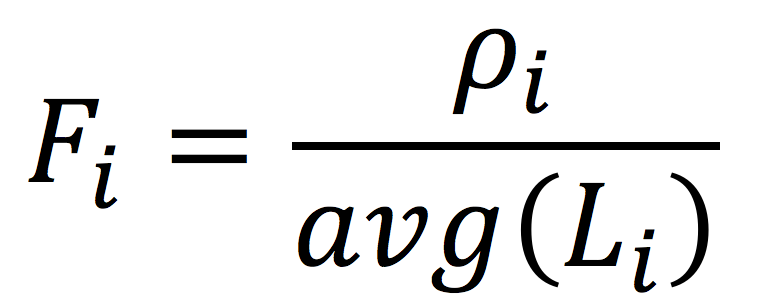# Use of Calibrated Reflectance Panels For MicaSense Data

Introduction

This article describes how to use a Calibrated Reflectance Panel (CRP) to relate raw pixel values from MicaSense images to absolute reflectance. Refer to the following articles as background information:

Calibrated Reflectance Panel and Calibration Information

Each CRP that MicaSense offers has a calibration curve associated with it across the visible and near-infrared spectrum. To obtain the calibration data for your panel, follow this link. The calibration data is provided as absolute reflectance (a value between 0.0 and 1.0) in the range of 400 nm to 850 nm (in increments of 1 nm). The calibration curve can be further simplified to be represented by 5 reflectance values, one for each of the 5 bands of the camera, by averaging the reflectance values in the calibration curve across the bandwidth of each band. See this link for definition of the center wavelength and bandwidth of a standard MicaSense camera.

Transformation of Raw Pixel Values Into Reflectance

To convert raw pixel values into reflectance, an image of the CRP taken before or after flight is used. A typical panel image (in this example for the NIR band) is shown below:To determine the transfer function, first convert the raw pixels of the panel image to units of radiance per the process specified in the radiometric calibration model article. Then calculate the average value of radiance for the pixels located inside the actual panel area of the image (the white square to the left of the QR code). The transfer function of radiance to reflectance for the ith band is:where:

• Fi is the reflectance calibration factor for band i
• ρi is the average reflectance of the CRP for the ith band (from the calibration data of the panel provided by MicaSense)
• avg(Li) is the average value of the radiance for the pixels inside the panel for band i

This factor can be used for the ith band to convert all radiance values to reflectance by simply multiplying the radiance values of any image by the factor Fi. This same process can be applied to each of the 5 bands independently to convert the images for each band to units of reflectance.

Note: In order to calculate a correct transfer function, it is critical that the image of the panel is not saturated. Select the image properly. And follow our guidelines for capturing images of the CRP.

Multiple Panel Images Across Time

If more than one panel image is captured (for example at the beginning and end of a flight) or if data for a field is captured in multiple flights and a calibration panel image is taken at the beginning of each flight, it is possible to extend the analysis above by interpolating across time.

All images captured by MicaSense sensors include date/time as part of their metadata (UTC reference). The date/time of the panel images can be used to define a time-varying model. This time-dependent model can then be applied to each image individually, taking into account the image's own date/time stamp. This can be achieved with a simple linear interpolation across CRP captures, or with a more complex least-squares fit model depending on the accuracy needed.